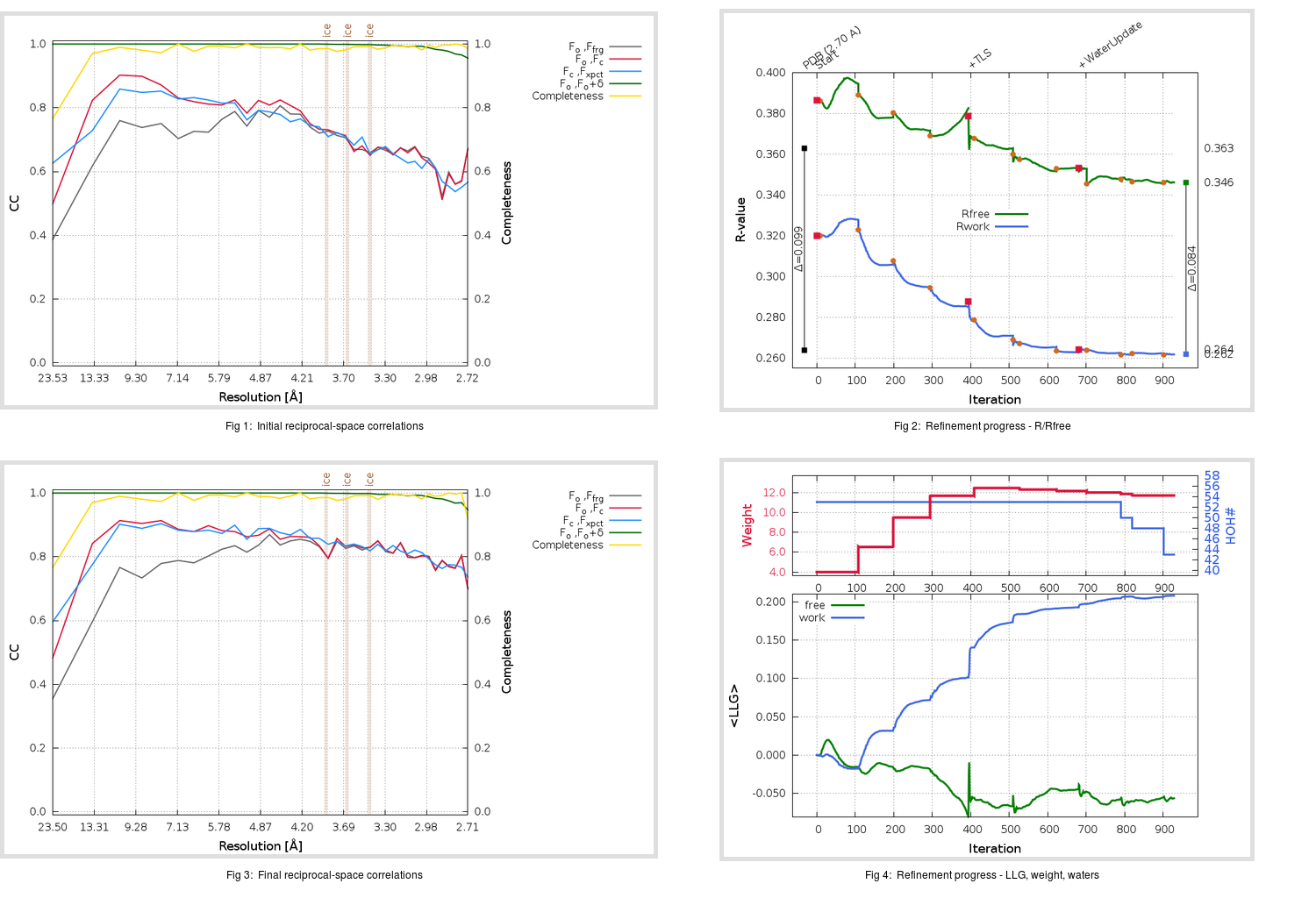Content:

```    Diffraction limits & principal axes of ellipsoid fitted to diffraction cut-off surface:
2.648         1.0000   0.0000   0.0000       a*
2.599         0.0000   1.0000   0.0000       b*
2.612         0.0000   0.0000   1.0000       c*
```

## Deposited

` `
 Date deposited Date data collection Resolution R, Rfree 20080518 20070607 2.70 0.2590 0.3630

Molprobity (CCP4 7.0 version) summary:

```Ramachandran outliers =   2.69 %
favored =  90.24 %
Rotamer outliers      =   6.98 %
C-beta deviations     =     0
Clashscore            =  12.33
RMS(bonds)            =   0.0084
RMS(angles)           =   1.09
MolProbity score      =   2.79
Resolution            =   2.70
R-work                =   0.2590
R-free                =   0.3630
```

```Number of waters      =    53

<B> (all atoms) =   37.01 ( sd =    5.45 ) for       2390 non-hydrogen atoms
<B>   (protein) =   36.68 ( sd =    4.85 ) for       2322 non-hydrogen atoms
<B>     (water) =   48.03 ( sd =   11.76 ) for         53 non-hydrogen atoms
<B>    (others) =   49.24 ( sd =    1.48 ) for         15 non-hydrogen atoms

B min/max       (all non-hydrogen atoms) =   24.21 /   74.53
B min/max   (protein non-hydrogen atoms) =   25.24 /   48.33
B min/max     (water non-hydrogen atoms) =   24.21 /   74.53
B min/max     (other non-hydrogen atoms) =   44.95 /   50.39
```

## BUSTER (re-)refinement

` `

Molprobity (CCP4 7.0 version) summary:

```Ramachandran outliers =   0.34 %
favored =  91.58 %
Rotamer outliers      =  10.47 %
C-beta deviations     =     0
Clashscore            =   9.52
RMS(bonds)            =   0.0113
RMS(angles)           =   1.61
MolProbity score      =   2.78
Resolution            =   2.70
R-work                =   0.2613
R-free                =   0.3460
```

```Number of waters      =    43

<B> (all atoms) =   62.60 ( sd =   15.69 ) for       2380 non-hydrogen atoms
<B>   (protein) =   62.76 ( sd =   15.74 ) for       2322 non-hydrogen atoms
<B>     (water) =   52.63 ( sd =   11.78 ) for         43 non-hydrogen atoms
<B>    (others) =   67.80 ( sd =    1.73 ) for         15 non-hydrogen atoms

B min/max       (all non-hydrogen atoms) =   28.67 /  111.19
B min/max   (protein non-hydrogen atoms) =   28.75 /  111.19
B min/max     (water non-hydrogen atoms) =   28.67 /   82.83
B min/max     (other non-hydrogen atoms) =   63.76 /   69.64
```

Refinement progression:Results:

` `
 File Remark 3D62_aB_refine.01_03_refine.pdb.gz exact refinement commands are in header 3D62_aB_refine.01_03_refine.mtz.gz including original deposited data and several re-refinement map coefficients 3D62_aB_refine.01_03_BUSTER_model.cif.gz including any non-standard compound restraints 3D62_aB_refine.01_03_BUSTER_refln.cif.gz